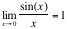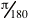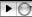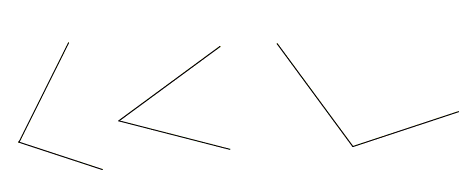P. Thompson

Extended Analysis of Functions 1

Fall 2005

Demonstration Lesson

Circular Functions

## What I am Teaching and What I Intend that Students Learn

I will teach the idea of radian measure. The foundation of this idea is angular measure as a fractional part of a circle's circumference. This idea of angular measure as a fractional part of a circle's circumference breaks into two parts:

 Strict fractional parts *     One degree = 1/360th of a circle's circumference *     One grad = 1/400th of a circle's circumference (German engineering unit) Linear measure of arc length *     One radian = arc that has a length equal to the circle's radius

The motivation for using radian measure in mathematics is this: The value of a trig function can be thought of as being a fractional part of a circle's radius. By having trig functions' arguments be in units of the circle's radius, a function's arguments and the function's values are in the same unit. (This is why the theorem "" is true only when x is in radians. When x is in degrees, this limit is.)

What I intend students to learn is summarized in my discussion of the activity sheet (activity sheets should be an expression of what you want students to learn). Briefly, I intend that they learn that measuring angles requires embedding angles within a circle and measuring the subtended arc, and that the best units of measure are in terms of proportional relationships (they will remain invariant across circles of different sizes). I also intend that they become skilled at estimating and approximating angles and their measures.

## Lesson Segment

I will scaffold the idea of angular measure as a measure of that part of a circle's circumference that the angle subtends.

In teaching this lesson, I assume students are familiar with protractors. I also assume that they will not understand a protractor as providing a measure of arc length. So, to make their idea of a protractor problematic (to them), I will start by asking them if, in principle, the size of the protractor matters when measuring an angle (within limits of practicality--it would be hard to imagine using a protractor having a radius of one mile).

Second, I will ask "Suppose we're making a protractor. We cut out a semi-circle--now, in principle, where do we put the degree marks?" This will raise the issue of just what is one degree (it is one three-hundred-sixtieth of any circle's circumference).

Third, I will ask "In principle, does it matter what size we make our circle?" In principle, no. Why? Because all circles are similar, so an angle having measure x degrees will always have measure x degrees no matter what size protractor we use. The measure will not be affected by shrinking or enlarging the circle. (I will use Sketchpad to demonstrate this, and ask the student to explain why the circle's size does not matter.)

Fourth, I will mention other conventional units (e.g., the "grad"), and unconventional but commonly used possibilities (e.g., "fifths"). I will also ask why we don't use, say, inches or centimeters to measure the arc length subtended by an angle. (Answer: Because changing the size of the circle would change the measure of any given angle while the angle itself would remain unchanged--and we want measures to be well-defined.)

Finally, I will introduce the radian as a unit of measure. I will use Sketchpad to suggest to students that any angle can be measured in multiple, equivalent ways, and that the measures are equivalent because they measure the same angle.

As I vary the position of L on the circle, I will ask the student to tell me what each of the measurements mean -- e.g., What does "Arc Length = 0.727 radii" mean? What does "cos(angle) = 0.747 radii" mean? Why is sin(angle) positive and cos(angle) negative as L passes through the second quadrant?

Click the PLAY button () to start this movie.

## Activity Sheet

I will give students pieces of string to use as compasses and ask them to make angles that measure 1 radian, 2 radians, 3 radians, 1.5 radians, 2.25 radians, and 3.75 radians. This part of the activity will give them an occasion to internalize the idea of radian measure of an angle.

The second part of the activity will ask students to get approximate measures of given angles, again using their string. This will give them occasions to develop a sense of magnitude for radian measures of angles.

The third part will ask students to relate common degree angle measures to their radian counterpart. This will provide them occasions to develop internal relationships among angle measures (e.g., since a full circle is 360 degrees and it is 2Pi radians,  then 90 degrees, being a quarter of the circumference, will be one-fourth of 2Pi radians, or Pi/2 radians).

# Activity Sheet

1.       Use a pen to mark a point on your string. Then draw a circle with the marked length of string as its radius. Use that one circle to do each of the following:

a)       Construct an angle having measure 1 radian.

b)      Construct an angle having measure 2 radians.

c)       Construct an angle having measure 3 radians.

d)       Construct an angle having measure 4 radians.

e)       Construct an angle having measure 5 radians.

f)       Construct an angle having measure 6 radians.

2.       Use a pen to mark a different point on your string. Then draw a circle with the marked length of string as its radius. Use the same point as the circle's center as you used in #1.

3.       Use your circle from #2 to do these:

a)       Construct an angle having measure 1.5 radian.

b)      Construct an angle having measure 2.25 radians.

c)       Construct an angle having measure 3.75 radians.

4.       Use your compass and your string to obtain an approximate radian-measure of these angles. Then use a ruler to get an approximate value for the sine and for the cosine of each angle. (See the introduction for a reminder of what "sine" and "cosine" mean.)a)       What is the radian measure of an angle having a degree measure of 360 degrees? Explain.

b)      What is the radian measure of an angle having a degree measure of 90 degrees? Explain.

c)      What is the radian measure of an angle having a degree measure of 72 degrees? Explain.

d)      What is the radian measure of an angle having a degree measure of 112 degrees? Explain.

e)      What is the radian measure of an angle having a degree measure of x degrees? Explain.

6.       Imagine a person sitting on a Ferris wheel as the wheel revolves around its center.

a)       Relate the idea of "how far that person has traveled" to the idea of arc length as an angle's measure.

b)      Describe that person's how we can keep track of this person's height above ground in relation to how far she has revolved around the Ferris' wheel's perimeter. Explain why that graph appears as it does.